# Reliability Tests

Central Semiconductor performs extensive reliability stress testing on a variety of products from our product portfolio. This data is collected in addition to Central's standard qualification test data for any new or changed product.

### Typical Results: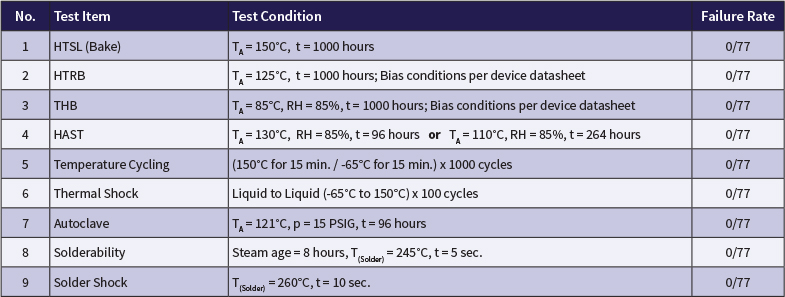# Reliability - FIT Rate (HTRB Life)

Data noted herein is based on standard FIT rate calculations as detailed below.

### FIT rate calculations: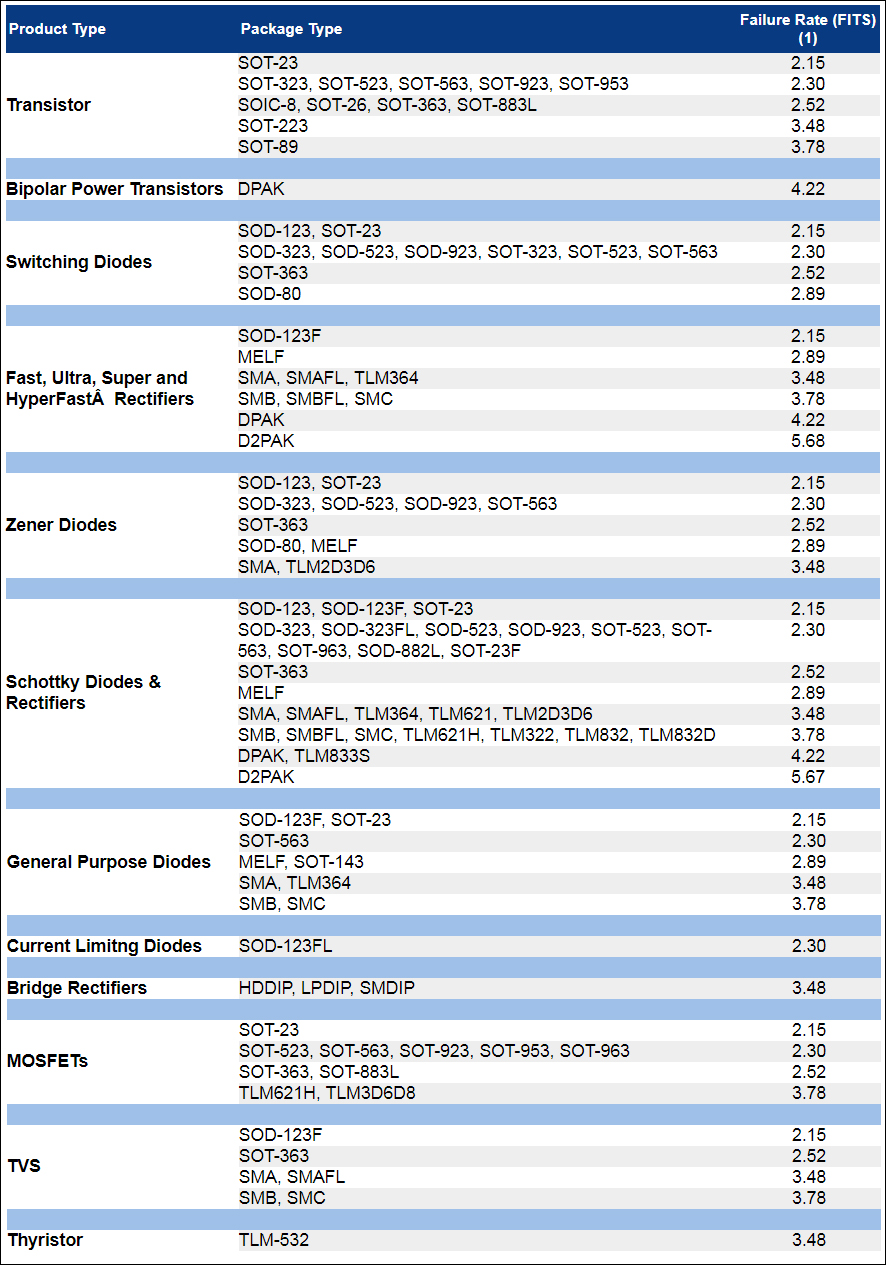(1) 60% Confidence Level. Accelerated testing.

# Formulas

The failure rate calculation based on a single life test is as follows: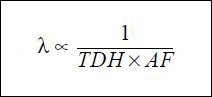λ = Failure Rate
TDH = Total Device Hours
AF= Acceleration Factor

The Accelleration Factor is calculated as follows: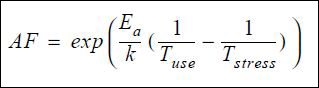Ea = Thermal Activation Energy
k =Boltzmann's Constant (8.63 x 10-5 eV)/K
Tuse= Use Temperature (°C + 273)
Tstress = Life test stress temperature (°C + 273)

The comprehensive failure rate is calculated as follow: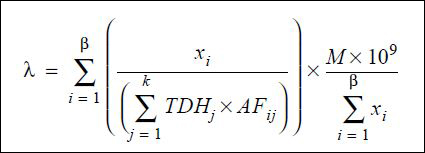β = Number of distinct possible failure mechanisms
k= Number of life tests combined
xi = Number of failures for a given mechanism (1 = 1, 2, ... B)
TDHj = Total device hours of test time for life testj (j = 1, 2,... k)
AFij = Acceleration factor for appropriate failure mechanism (i = 1, 2,... k)
M = X² (∝ , 2r +2) / 2
(where X² = chi square factor for 2r +2 degrees of freedom, r = total number of failures, ∝ = risk associated with CL between 0 and 1)

# Package Reliability Summaries

Reliability Summaries for surface mount and through-hole package types can be found in the "Package Reliability" column of the tables below.

## Surface Mount Packages

Package Type
Package Details
Material Composition
Package Reliability
LPDIP (MICRO DIP)
SOD-323FL (MICRO SMP)
SOD-882 (X1-DFN1006-2)
SOD-882L (X2-DFN1006-2)
SOIC-16 (SO-16)
SOT-143 (TO-253AA)
SOT-523W (SC-75)
SOT-883L (X2-DFN1006-3)
SOT-883VL (X3-DFN1006-3)
TLM1031 (UDFN10)
TLM2D3D6 (DFN0603-2)
TLM3D6D8 (DFN0806-3)
TLM322 (UDFN2020-3)
TLM322S (UDFN2020-3)
TLM364 (TO-277A)
TLM621 (DFN2010-6)
TLM621H (DFN2015-6)
TLM832 (UDFN3020-8)
TLM832D (UDFN3020-8B)
TLM832DS (UDFN3020-8B)
TLM833 (UDFN3030-8)
TLM833S (UDFN3030-8)

## Through-Hole Packages

Package Type
Package Details
Material Composition
Package Reliability